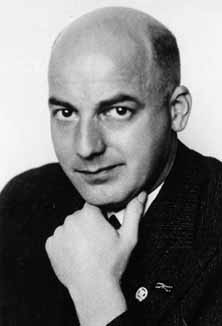# How many fields share a common discriminant?

## The multiplicity problem

What is the meaning of the discriminant?

The concept of discriminants is derived from the latin word "discriminare", which means "to distinguish". Until the first quarter of the 20th century it was believed that algebraic number fields with a fixed signature and Galois group can be identified uniquely up to isomorphisms by the integer value of the discriminant of their maximal order. In this case, non isomorphic fields could be distinguished uniquely by the discriminant.

For example, even the famous mathematician E. ARTIN conjectured at about 1925 that it be possible to show, using class field theory, that nonisomorphic fields never have coinciding discriminants. This is indeed true for quadratic fields.

The katastrophe:

However, it was nothing else but class field theory, which provided the first counter examples for this conjecture in a fundamental work of A. SCHOLZ and O. TAUSSKY  at about 1930 in the form of some families of 4 non isomorphic complex cubic fields with the same discriminant d, e. g. d = -3299, -3896, -4027, -5703. These and numerous further examples are also investigated in my paper 

Generally we call the number of non isomorphic fields with a fixed signature and Galois group sharing a common discriminant d the multiplicity m of the discriminant d.

Later, the construction of increasingly extensive tables of cubic fields of the signatures (1,1) and (3,0) with the aid of main frame computers revealed further examples of families with higher discriminantal multiplicities m = 2,3,4,6,9. Some errors in such tables caused a lot of confusion concerning the multiplicity m = 5. Recently, even quintic fields with m = 2 have been discovered.

Begin of a theory of multiplicities:Two fundamental methods of deriving exact formulas for the multiplicity m of cubic discriminants are due

1. to H. HASSE (1930) with the aid of class field theory of the associated normal fields 
2. to H. REICHARDT (1933) by means of Kummer theory of the associated radical fields 

The access of REICHARDT can essentially be recognized already in 1925 in a paper of W. E. H. BERWICK  and has been developed further by J. MARTINET and J.-J. PAYAN  ,  in 1965.

Current theory of multiplicities:

In 1990 I have generalized the idea of HASSE for dihedral fields of degree 2p with a prime number p > 2  and in 1991 together with Pierre BARRUCAND at Paris for pure metacyclic fields of degree p(p-1) . The result was a huge number of new formulas for the multiplicity m in dependence on invariants of associated quadratic fields k, such as:

D. C. MAYER's formulas:

 m = pr (p-1)u [(p-1)v-1 - (-1)v-1] / p m = pr+w (p-1)u [(p-1)v-1 + (-1)v-a(1)(p-1)a(1) + ... + (-1)v-a(n)(p-1)a(n)] / p2

Here, r denotes the p-class rank of k, t, u, and v respectively the number of all, of the free, and of the restrictive prime divisors of the conductor, w = 0,1 the indicator for the irregular prime divisor 3 of the conductor, n = p+1 the number of hyperplanes of a 2-dimensional p-vectorspace, and a(1),...,a(n) the partition of v into the numbers of prime divisors of the conductor associated with the hyperplanes. Some applications for the cubic case p = 3 are given by 3-networks over quadratic number fields with 3-class rang r = 2.

Formulas of P. BARRUCAND and D. C. MAYER:

 m = (p-1)t m = (p-1)u [(p-1)v - (-1)v] / p m = (p-1)u [(p-1)v-1 - (-1)v-1] / p

For the most recent results see my series Vienna Congress 2001.

Bibliography: